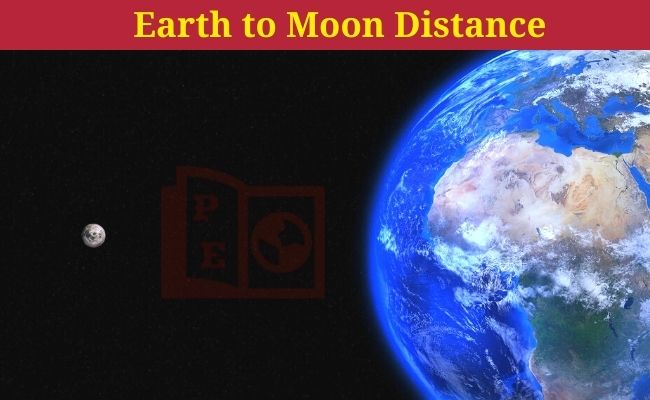# Earth to Moon Distance (in km, miles, AU, and light-years)Moon is the Earth’s only natural satellite. The moon orbits the earth in around 27.32 days. And the average distance from the Earth to Moon is around 384400 kilometers. But the orbital path of the moon around the earth is not a perfect circle, though it varies. So, according to the orbital path, the actual Earth to Moon distance varies every moment.

## Actual Earth to Moon Distance

In actuality, the distance between the moon and earth always changes. Because our moon does not orbit Earth in a perfect circle but the path is a little elliptical. Also, according to Lunar Laser Ranging (LLR) experiment, scientists have concluded that the moon is drifting away around 3.8 cm in one year from the earth. So, there is always variation between the earth and moon’s actual distance. At some point, the moon is closest to earth and at some point in the orbital path, the moon would be the farthest from the earth. The closest distance is called perigee, whereas the farthest distance between the moon and earth is known as apogee.

Here in this article, we will know about the mean or average distance of the moon from earth. Also, closest ( perigee) and farthest (apogee) distances are given. We will know how far is away is the moon from the earth in many different units, like kilometers, miles, astronomical units (AU), and light-years (light-seconds).

### Earth-Moon distance in kilometers (km)

• The average Earth-Moon distance is 384400 km.
• Closest distance -: 362600 km.
• Farthest distance -: 405400 km.

### Moon distance from Earth in Miles

• The moon is 238855 miles away from the earth.
• Closest distance -: 225309 miles.
• Farthest distance -: 251904 miles.

### Distance from Earth to Moon in AU

• In Astronomical Unit, the average distance of the moon from the earth is 0.0025695 AU
• Closest distance -: 0.002424 AU.
• Farthest distance -: 0.002710 AU.

### Earth-Moon distance in light-years (light-seconds)

One light-year is around 9.46 × 1012 km. This is the unit of length, used for measuring long astronomical distances. Whereas one light-second is around 300,000 km (light traveled in one second).

• The average distance of the earth from the moon is around 1.282 light seconds or 4.063 × 10-8 light-years.
• Closest distance -: 1.2095 light seconds.
• Farthest distance -: 1.3522 light seconds.

So, these were the distance between the earth and moon in many units.

Also Check:-